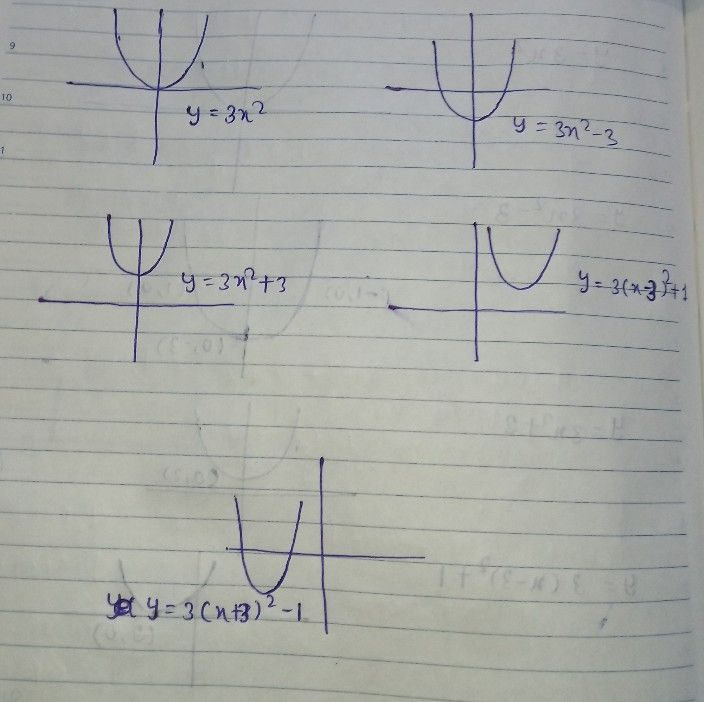Symbol
Problem$4$ $x$ Learning Task $4$ Illustrate the graphs of the following quadratic functions then analyze the effects from each other. $1.y=3x^{2}$ $3$ $2.y=3x^{2}-3$ $3.y=3x2+3$ $-7$ 6 -5 $3$ $-2$ $4.y=3\left(x-3\right)^{2+1}$ $5.y=3\left(x+3\right)^{2-1}$
7th-9th grade
Algebra
Search count: 108
SolutionQanda teacher - priyamamun